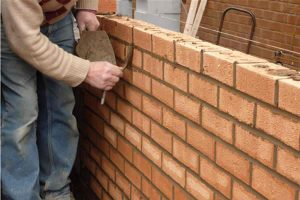## How many bricks are required for 1 cubic meter? | How much mortar quantity required for 1 cubic meter?We are going to explain here how to calculate the number of bricks required for 1cum and how much mortar is required for 1 cum.

500 no. of bricks (brick size – 20cm.*10cm.*10cm.) required for 1 cum of brick masonry.
and suppose, ratio = 1:6
then, sum = 1+6 = 7

We use 30% mortar in brick work, So 1 cum of brickwork has 0.3 cum of mortar. Quantity for 1:6 mortar mix
as we know, total dry mortar required for 1 cum. of brick masonry = 0.30 cum.

Therefore following dry material required.
Cement = 1* 0.30/7 = .04 cum. *(1/0.0347) = 1.15 bags.

Density of cement = 1440Kg/cum
So 1 bag of Cement Density=50/1440 = 0.0347Kg

Sand = 6* 0.30/7 = 0.26 cum.
or 0.26 cum. * 35.3 = 9.18 cft.
Material required for 1 cum. of brick masonry in mortar
ratio of 1:6.
Cement = 1.15 bags.
Sand = 9.18 cft.
Bricks = 500 nos.